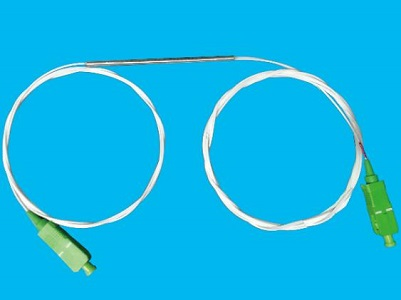### Current position：Home / Products

 Thermal Expanded Single Core FiberProduct Features Product Applications Low Excess Loss Low Loss Splicing Point for Different Fibers Large Expanded Field Stable Fiber Alignment More Tolerance for Alignment High Power Connector Pigtails High Coupling Efficient to Laser
 Specifications Parameter Unit Excess Loss Max dB 0.1 Excess Loss Typ dB 0.04 Expanded Core Length (Le) Min mm 2.0 Available Polishing Length Min mm 0.5 Mode Filed Diameter Increase Typ % 100 Glass Fiber Length (Lg) Min mm 8

 Typical SpectrumOrder information T E C Mode Filed 1=100% 2=200% Glass Length, Lg 04=4mm 05=5mm 06=6mm 07=7mm ….. 20=20mm Expand Length, Le 02=2.0mm 03=3.0mm 04=4.0mm ….. 10=10.0mm Fiber Type 1=G652 or Equivalent 2=HI1060 3=HI1060 FLEX 4=980-20 5=980-16 6=HI780C 7=Panda1550 8=Panda980 9=Panda780 Pigtail S=250μm bare fiber M=0.9mm Loose tube L=3mm Cable Fiber Length 0=0.5m 1=0.75m 2=1.0m 3=1.5m S=Specify Connector (TEC fiber) 0=None 1=FC/PC 2=FC/SPC 3=FC/APC 4=SC/SPC 5=SC/APC 6=ST 7=FC/UPC 8=SC/UPC 9=MU A=LC/PC B=SC/PC C=LC/UPC D=LC/APC E=FC Ferrule F=LC Ferrule G=FA Connector (Normal fiber) 0=None 1=FC/PC 2=FC/SPC 3=FC/APC 4=SC/SPC 5=SC/APC 6=ST 7=FC/UPC 8=SC/UPC 9=MU A=LC/PC B=SC/PC C=LC/UPC D=LC/APC E=FC Ferrule F=LC Ferrule G=FA

Note: All specifications are before connectors and are subject to change without notice.